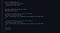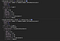# 10 Javascript Design Patterns To Improve Your Code With

## Factory Pattern

`const express = require('express');const app = express();`Code from Express GitHub

## Observer Pattern

`function Observable(observer) {  this.subscribe = (...fns) => {    if(observer) {      const obs = new Observer(...fns);      observer(obs);    }  };    this.unsubscribe = () => {    observer = null;  }}`
`function Observer(  onNext,   onError = () => {},   onComplete = () => {}) {  const def = () => {};  if (typeof onNext === 'object') {    onError = onNext.error || def;    onComplete = onNext.complete || def;    onNext = onNext.next || def;  }  this.completed = false;  this.withError = false;  this.next = (val) => {    if (!this.completed) onNext(val);  };  this.error = (err) => {    if (!this.completed) {      this.completed = true;      this.withError = true;      onError(err);    }  };  this.complete = () => {    if (!this.completed) {      this.completed = true;      onComplete();    }  };}`
`const obs = new Observable((observer) => {  observer.next(10);  observer.next(20);  observer.complete(new Error());  observer.error(new Error());})obs.subscribe({  next(value) {    console.log('next', value);  },  error(err) {    console.log('err', err);  },  complete() {    console.log('complete');  },});obs.unsubscribe();`

## Module Pattern

`(function(require, global, exports, module, __dirname, __filename) {  // your Node code goes here})();`
`const globalData = {  x: 20};const myModule = (function(global) { // <- access injections  // private stuff in the module  const val = 10 + global.x;  // expose what you want  return {    prop: 12,    method() {      return val;    }  }})(globalData); // <- inject into your moduleconsole.log(myModule.prop); // prints 12console.log(myModule.method()); // prints 30`

## Proxy Pattern

`let validator = {  set: function(obj, prop, value) {    if (prop === 'age') {      if (!Number.isInteger(value)) {        throw new TypeError('The age is not an integer');      }      if (value > 200) {        throw new RangeError('The age seems invalid');      }    }    // The default behavior to store the value    obj[prop] = value;    // Indicate success    return true;  }};const person = new Proxy({}, validator);person.age = 100;console.log(person.age); // 100person.age = 'young';    // Throws an exceptionperson.age = 300;        // Throws an exception`

## Facade Pattern

`// facade around the XMLHttpRequest API// with support for Promise and POST // JSON and Multipart Dataconst request = (() => ({  post(url, data = {}) {    const req = new XMLHttpRequest();    if(data instanceof File) {      formData = new FormData();      formData.append('file', data, data.name);      data = formData    } else {      data = JSON.stringify(data);    }    req.open('POST', url, true);    return new Promise((res, rej) => {      req.onload = (e) => {        if (req.status === 200) {          res(e);        } else {          rej(e);        }      }      req.onerror = rej;      req.onabort = rej;      req.onabort = rej;      req.ontimeout = rej;      req.send(data);    });  },  get() {    // code here  }}))();request.post('https://www.domain.com/api/endpoint', {some: 'data'})  .then(e => {    console.log('success', e)  })  .catch(e => {    console.log('failed', e)  })`

## Iterator Pattern

`// simple iterator that takes a // function to get the next value// which is called on every iteration// and completes if the function// returns nullfunction Iterator(getNextValue) {  this.next = () => {    const value = getNextValue();        if(value === null) {      return {done: true};    }        return {value, done: false};  };    this[Symbol.iterator] = function() { return this; }}`
`const thousandList = {};// using generator to implement an iteratorthousandList[Symbol.iterator] = function* () {    let number = 0;    yield number;    while(number < 1000) {      yield ++number;    }};for(const number of thousandList) {  console.log(number); // prints 1 to 1000}[...thousandList] // [1, 2, 3, ..., 1000]`

## Prototype Pattern

`function Calculator() {  // total is public because we declared it on the "this"  this.total = 0;  // precision is private because is a local variable/constant  const precision = 2;  // to precision is a public function expression with access to   // private properties  this.toPrecision = (number) => Number(number.toFixed(precision));    // create a getter for the property "result"    Object.defineProperty(this, 'result', {    get() {      return this.total;    }  })}// create a static member// only available on Calculator. It cannot be inheritedCalculator.PI = 3.14;// prototype methodsCalculator.prototype.add = function(x) {  this.total += this.toPrecision(x);}Calculator.prototype.subtract = function(x) {  this.total -= this.toPrecision(x);}Calculator.prototype.multiply = function(x) {  this.total *= this.toPrecision(x);}Calculator.prototype.divide = function(x) {  this.total /= this.toPrecision(x);}function ScienticCalculator() {  // this is equivalent to calling super()   // when you extend another class  // it will copy all properties from inside Calculator   // into ScienticCalculator  // You can call as many constructor functions  // to inherit properties from multiple ones  Calculator.call(this);}// make ScienticCalculator extend Calculator// similar to what happens when you do // "class Calculator extends ScienticCalculator"ScienticCalculator.prototype = Object.create(Calculator.prototype);ScienticCalculator.prototype.constructor = ScienticCalculator;const calc = new Calculator();const scientificCalc = new ScienticCalculator();console.log(calc);console.log(scientificCalc);// checks logs below`Code on CodePen

## Decorator Pattern

`// An example with no decorator Support// through Object Augmentationfunction logger(obj, prop, message = 'logger') {  let x = obj[prop];      if(typeof x === 'function') {    obj[prop] = (...args) => {      console.log(message, ...args);      x.call(obj, ...args)    }  } else if(obj.hasOwnProperty(prop)) {    Object.defineProperty(obj, prop, {      get() {        console.log(message + ' - get:' , x);        return x;      },      set(val) {        console.log(message + ' - set:', val);        x = val;      }    })  }}class Calculator {  total = 0;    add(x) {    this.total += x;  }}const calc = new Calculator();logger(calc, 'total', 'total');logger(calc, 'add', 'add argument');calc.add(20);// logs// add argument: 20// total - get: 0// total - set: 20`

## Conclusion

Blog & YouTube Channel for Web, UI & Software Development - beforesemicolon.comyoutube.com/c/BeforeSemicolon

## More from Before Semicolon

Blog & YouTube Channel for Web, UI & Software Development - beforesemicolon.com — youtube.com/c/BeforeSemicolon